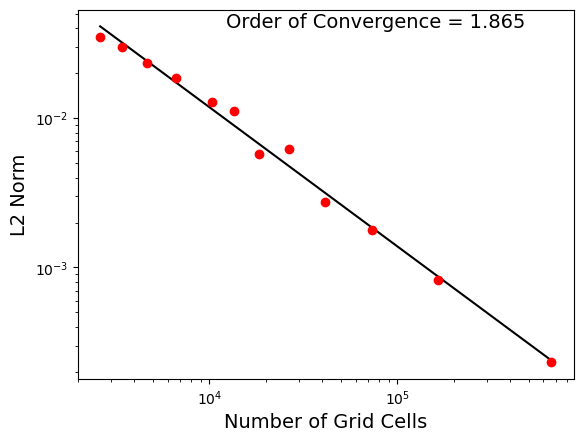# global_convergence¶

The global_convergence test group implements convergence studies on the full globe. Currently, the only test case is the advection of a cosine bell.

## cosine_bell¶

The cosine_bell test case implements the Cosine Bell test case as first described in Williamson et al. 1992 but using the variant from Sec. 3a of Skamarock and Gassmann. A flow field representing solid-body rotation transports a bell-shaped perturbation in a tracer $$psi$$ once around the sphere, returning to its initial location. The bell is defined by:

$\begin{split}\psi = \begin{cases} \left( \psi_0/2 \right) \left[ 1 + \cos(\pi r/R )\right] & \text{if } r < R \\ 0 & \text{if } r \ge R \end{cases}\end{split}$

where $$\psi_0 = 1$$, the bell radius $$R = a/3$$, and $$a$$ is the radius of the sphere. The equatorial velocity $$u_0 = 2 \pi a/ (\text{24 days})$$. The time step is proportional to the grid-cell size.

By default, the resolution is varied from 60 km to 240 km in steps of 30 km. The result of the analysis step of the test case is a plot like the following showing convergence as a function of the number of cells:### config options¶

The cosine_bell config options include:

# options for cosine bell convergence test case
[cosine_bell]

# the number of cells per core to aim for
goal_cells_per_core = 300

# the approximate maximum number of cells per core (the test will fail if too
# few cores are available)
max_cells_per_core = 3000

# time step per resolution (s/km), since dt is proportional to resolution
dt_per_km = 30

# the constant temperature of the domain
temperature = 15.0

# the constant salinity of the domain
salinity = 35.0

# the central latitude (rad) of the cosine bell
lat_center = 0.0

# the central longitude (rad) of the cosine bell
lon_center = 3.14159265

# the radius (m) of cosine bell

# hill max of tracer
psi0 = 1.0

# time (days) for bell to transit equator once
vel_pd = 24.0

# convergence threshold below which the test fails for QU meshes
qu_conv_thresh = 1.8

# Convergence rate above which a warning is issued for QU meshes
qu_conv_max = 2.2

# convergence threshold below which the test fails for icosahedral meshes
icos_conv_thresh = 1.8

# Convergence rate above which a warning is issued for icosahedral meshes
icos_conv_max = 2.2


The last 7 options are used to control properties of the cosine bell and the background properties. The first 4 options are discussed below.

### resolutions¶

The default resolutions used in the test case depends on the mesh type. For the qu mesh type, the defaults are:

resolutions = 60, 120, 240, 480


for the icos mesh type, they are:

resolutions = 60, 90, 120, 150, 180, 210, 240


To alter the resolutions used in this test, you will need to create your own config file (or add a cosine_bell section to a config file if you’re already using one). The resolutions are a comma-separated list of the resolution of the mesh in km. If you specify a different list before setting up cosine_bell, steps will be generated with the requested resolutions. (If you alter resolutions in the test case’s config file in the work directory, nothing will happen.) For icos meshes, make sure you use a resolution close to those listed in Spherical Meshes. Each resolution will be rounded to the nearest allowed icosahedral resolution.

### time step¶

The time step for forward integration is determined by multiplying the resolution by dt_per_km, so that coarser meshes have longer time steps. You can alter this before setup (in a user config file) or before running the test case (in the config file in the work directory).

### cores¶

The number of cores (and the minimum) is proportional to the number of cells, so that the number of cells per core is roughly constant. You can alter how many cells are allocated to each core with goal_cells_per_core. You can control the maximum number of cells that are allowed to be placed on a single core (before the test case will fail) with max_cells_per_core. If there aren’t enough processors to handle the finest resolution, you will see that the step (and therefore the test case) has failed.